# Pharma Engineering

An Engineer's Choice

• ## Wednesday, 14 September 2016

Hello guyzzz..........!!!

Back after many months and sorry for not being regular here, i've received a query from one of the visitor, and i think many of you too have the same question i.e., How to calculate the rate of distillation in a batch reactor....??

And for this query today i gonna put a end mark, i'll explain you with an example here,
but, before that you need to know some basics like, when are we going to do distillation in a batch reactor during a product manufacturing......!!
Most commonly there are 2 cases,

1) after completion of reaction, for impurity removals we will do some workups with solvents like water, which is followed by product layer concentration, simply called as product concentration,

2) The 2nd one will be removal of Moisture from the raw materials, for this the raw materials should be made soluble in a suitable solvent and then the solvent medium is distilled off under vacuum, which will remove the water molecules from the raw materials and make them suitable for carrying out reaction, this is usually done for moisture sensitive products.

So, here now i'll demonstrate an example case study,

Recommended Articles:

How To Design a Condenser

Our case is, we need to calculate the distillation rate in a reactor having a product layer of Ethyl Acetate, and the distillation should be carried out with vacuum NLT 650 mmHg, and temperature NMT 40 deg C,

Let suppose our reactor capacity is 10 KL with MOC SS316, and the product layer volume is around 5 KL say,

Now we need to collect some raw data like HT area of SSR, Specific heat & Density of Solvent, etc.

10 KL SSR will have an HT area of 19.82 Sq.m, but the effective HT area for this operation will be equals to Total HT area x Occupancy of reactor,

Occupancy of reactor = Reaction mass volume / Reactor volume = 5 KL / 10 KL = 0.5,

Effective HT Area = 19.82 x 0.5 = 9.91 Sq.m,

[How To] Calculate Reactor HT Area

Density of Ethyl Acetate is 897 Kg/cu.m, Specific heat : 0.454 KCal/Kg. K , Latent Heat of vaporisation is 87.6 KCal/Kg,

As the MOC of the reactor is SS316, the Overall Heat Transfer Coefficient can be considered as 250 KCal/Sq.m. Hr. K.

If you want to calculate the Overall Heat Transfer of a reactor with more accuracy, then read this post,

[How To] Calculate Overall Heat Transfer Coefficient

So now, getting into our calculation we need to calculate the amount of energy that our HT area is going to transfer per hour, and that we can calculate as follows,

Q = U x A x LMTD,

Sorry guys, i forgot to calculate the LMTD previously, anyway now i'll do it for you,

for calculating the LMTD we need two streams, one is utility stream and the other one is batch volume,

So , coming to Hot water utility, now you may have one doubt that why i've used Hot water as utility, the reason is we have a Specification that reaction mass temperature shouldn't exceed 40 deg C, thats why i've considered Hot water instead of Steam, and now another question may arise in your mind, what can be the hot water set point, usually we will maintain a Delta T of 10 deg C, so the Hot Water Set point will 40 deg C + 10 = 50 deg C.

Recommended Articles:

How to Select a Vapour Column
Pumps in Series Vs Pumps in Parallel

so now Th1 = 50 deg C, Th2 = 45 deg C (say), Trxn = 25 deg C [ i've considered the reaction mass temperature as 25 deg C because at 650 mmHg of vacuum, the boiling point of ethyl acetate will be hardly 25deg C, this can be calculated from antonie equation],

LMTD = ( ( Th1 - Trxn ) - ( Th2 - Trxn ) ) / Ln [ ( Th1 - Trxn ) / ( Th2 - Trxn ) ]
=  ( ( 50 - 25 ) - ( 45 - 25 ) ) / Ln [ ( 50 -25 ) / ( 45 - 25 ) ]
=  5 / Ln [ 1.25 ] = 22.727 Deg C,

Now, lets resume our calculation,

Q = U x A x LMTD

=  250 x 9.91 x 22.727 = 56306.143 KCal.

Now we need to know what is the Heat load of the Reaction mass i.e., summation of Sensible heat & Latent heat,

Qhl = Sensible heat + Latent heat

=  M x Cp x dT   +   M1 x Lambda

= ( V x Rho )  x Cp x dT   + M1 x Lambda

=  5 x 897 x 0.454 x ( 30-25)  +  M1 x  87.6

=  10180.95 + M1 x 87.6

Now, we need to equate the overall heat load to the reactor supply load i.e., 56306.143 Kcal,

10180.95 + M1 x 87.6  =  56306.143

M1 x 87.6  =  46125.192

M1 = 526.54 Kgs of Ethyl Acetate = ( 526.54 / 0.897 ) Lts of ethyl acetate

M1 =  587 Lts of Ethyl Acetate.
If you guys understood the above done calculation clearly, Then say Cheers :)

Related Articles:

How To Design a Chiller Plant
How to Calculate Energy of Steam ?
How to select a Vacuum pump ?
How to Calculate Volume occupied by Torispherical Dish ?Hi! I am Ajay Kumar Kalva, Currently serving as the CEO of this site, a tech geek by passion, and a chemical process engineer by profession, i'm interested in writing articles regarding technology, hacking and pharma technology.

1.You cannot equate Q with Qhl as unit of Q is Kcal/Hr and not Kcal

1.ok, but can you tell me what are the units for U.....??

And for your king information Qhl will also be having KCal/hr only as the variable M in eqn of Qhl is having the units of Kg/Hr, and this whole posts describes about rate of distillate per hour, so the value of time is taken as unity and anything multiplied with unity returns the same, thats why i've neglected that Hr in whole post.

Still any queries, feel free to comment

2.Please explain LMTd a bit clear example.
Here im giving the e.g,
EA distillation T<25°C,Hotwter as medium
Here Temp of hot body will be hot water and cold body will be Rxn mass(10°C)
So why cant we make difference of (Temp of reactor- Temp of jacket) instead of lmtd.
Why should we use LMTD
Xplain bit clearly.
2streams are existing,but pls clearly justify me wid a xample

1.If you consider the difference between reaction mass and utility, then the answer will be a crap one, because whenever you need to calculate the energy of a stream then you need to consider both temperatures of that stream only, never mess with temperatures of two different streams, unless it is a LMTD, LMTD is used while calculating overall heat transfer rate

3.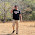You are taken 5 kl but 500 kgs out.....im not understood

1.yup, i've taken 5KL and i got 526 Kgs, thats mass flowrate, if u need in Lts then divide the 526 with solvent density.

2.526 lts for 1hr or what??
If in 5KL, EA is 2KL how much time it will take to distill off completly

3.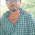Dear ,

Reactor capacity is 10 KL and in that 5KL of EA is considered, so out of that we can distill out 587 L of EA per hour(1st hour), pl dont get confused w.r.t. units, its 526 Kgs of EA which is equivalent to 587 Lts of EA.

Once again reminding you pl dont get confused.

Best Regards,
AJAY K

4.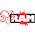I getting confusion about using LMTD and DT. When we should use LMTD and DT??

1.whenever you calculate heat load of a single fluid then then you may go with dT, but whenever the notation overall heat transfer coefficient arrives, then automatically LMTD should be used as there will be two fluids involving at that time

Regards,
PHARMA ENGINEERING

5.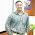But latent heat of ethyl acetate under vacuum will change.. Can u calculate that latent heat and then rate of distillation.. Eg. If u take ethyl acetate and heat under atmospheric condition and in another heat under vacuum... Boil up will increase under vacuum due to change in latent heat..

1.that major difference between boil up is due to relative lowering of vapour pressure, and the latent heat will also have some impact for sure, but not that much....... simply below limit of consideration.

Regards,
Ajay Kumar

6.can you cal the distillation time required to distill out 587 lits of EA for the same conditions you have explained.

7.I want the distillation rate per hr. not the quantity to be distilled....\

8.it means 587 lits of EA/hr???

9.What ever the 587Lts i've derived indicates the distillation rate but not the qty to be distilled

10.I am getting confusion in sensible heat part of your heat load calculation....why you have taken (30-25) as delta T....since rxn mass is already at boiling point did'nt we have to just consider latent heat part??

1.Hey buddy, i've considered that the distillation is happening in RT, so i've considered 30 degC, and the Rxn mass is at 25 degC, so in order to raise the temp of rxn mass to 30degC from 25 degC, the sensible heat is required, and also the sensible heat load will be far low than the latent heat when compared, if required you can neglect it.

2.Liquid Ammonia boiling point is -33.34 degC.

3.I cant understand sensible heat part of your heat load calculation....why you have taken (30-25) as delta T...please clarify

4.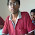Hey, that 30-25 means, your reaction mass is at RT, and that reaction mass temperature should be raised upto 30 degC from 25 degC, then under vacuum it will start boiling, thats why i've considered it as sensible ehat load, if required you can ignore that part.

Regards,
AJAY K

11.is it applicable for multi component mixture

1.Applicable but if the required distillate is a low boil.

Regards,
AJAY K

12.What is the necessity to calculate sensible heat after calculating Q value

1.Dear Naveen,

Sensible heat is always a part of heat energy required, it may be very less when compared to latent heat, but it cant be neglected.

Regards,
AJAY K

13.hey Ajay during distillation your heat transfer Area will change. so you have to calculate for different area ...

1.Dear Nitin,

For sure, the effective heat transfer area will change, also the rate of distillation will decrease. This is just a general calculation.
If we need to calculate the time for distillation, we need to reduce the mass distilled out from the reaction mass, and then the effective heat transfer area shall be calculated.

Regards,
AJAY K

14.What is rho here

1.Dear Naveen,

rho means density.

Regards,
AJAY K

15.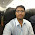My name is Kinjangi BalaRaju

Dear Ajay sir, why don't u use like this Q=M(Cp x dT + Lambd).PLEASE Explain sir.this is applicable to all or not.which is better to use.please reply sir

1.Hii bala,

Usually we wont distill out all the mass, if we need to distill out all the available mass then we can use the formula as like you mentioned,
but we need to heat the total reaction mass in order to start distillation,

Hope you understand, if any further queries, feel free to comment here / message me from contact me page.

Best Regards,
AJAY K

16.Value of U for MSGL reactor ??

1.That's slightly based on the utility we provide to the jacket,
But as per thumb rule we will consider it as 100-150 KCal/hr.Sq.m.K.

Regards,
AJAY K

17.Sir little confusion ,if you take it heated from 25C to 30C during calculation of sensible heat , then why you not take it in the Calculation LMTD, you take only 25C insted of 30c.

18.Hi Ajay...
If we use steam instead of hot water, what will be the inlet and outlet temperatures of reactor jacket... As per your experience for above case study....
Regards,
Philip

1.Dear Philip,

If you are going to use steam, the inlet depends on the pressure of steam and for that pl refer the steam tables, https://www.pharmacalculations.com/2018/04/steam-tables.html,
And for the steam usually the outlet would be the same as like inlet, there wont be much difference, if the inlet is 110 C, then the outlet would also be ~108-110 C. As most of the times we would like to use the latent heat of steam only but not sensible heat.

Best Regards,
AJAY K

19.Hi Ajay,

You have explained the concept very neatly.
I'm a process engineer.
Most of the time, we assumes the values which we cant measure or to fit the values in given formula.
In the above example we've assumed 3 values: OHTC, Hot water outlet temperate Rh2 & Mass heating temperature from 25 to 30 Deg. C
How we can justify these assumptions to non technical persons who arises the question about these assumptions.

1.Hiii ,

Overall heat transfer coefficient is calculated based on individual heat transfer coefficients, thermal conductivity and thickness of the surface.
Again Individual heat transfer coefficients depends on the thermal conductivity, reynolds number and prandtl no.
Again prandtl no. depends on thermal conductivity.
So, as per me OHTC depends majorly on thermal conductivity of the metal.
For SS316 it will be in range of 13-17 w/m.K, for MSGL surface it will be in range of 5-8 w/m.k, for hastelloy it will be in range of 30-35 w/m.k.

Based on this we will assume the OHTC, as for SS316 it will be around 250-300 KCal/m2.hr.C, For MSGL it will be 100-150 KCal/m2.hr.C and hastelloy it will be 300 + ,
Based on the composition as there will be many types in hastelloy.
Second thing is hot water outlet temperature, as per my practical experience i'm telling you that the difference between the inlet and outlet would be less than 4 C, but as thumb we'll take it as 5 C. if required we can show them(Non-technical persons) practically.
And lastly, as we are distilling out the mass below 30 C, i've taken it as worst case i.e., ambient temperature as 25 C and the difference is between 30-25 = 5 C.

Next time, kindly comment with your good name plzz.

Best Regards,
AJAY K

2.And one more thing to add, the OHTC's we have considered will be average or worst case, they might be higher than we have taken. for evaluating those we need to take trials and it will vary from fluid to fluid.

Best Regards,
AJAY K

3.Thanks for the clarification.Hi! I am Ajay Kumar Kalva, Currently serving as the CEO of this site, a tech geek by passion, and a chemical process engineer by profession, i'm interested in writing articles regarding technology, hacking and pharma technology.